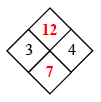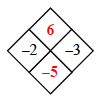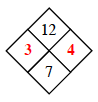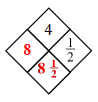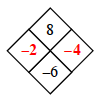### Home > CC3 > Chapter 1 > Lesson 1.1.1 > Problem1-4

1-4.

DIAMOND PROBLEMS

Finding and using a pattern is an important problem-solving skill you will use in algebra. The patterns in Diamond Problems will be used later in the course to solve other types of algebraic problems.

Look for a pattern in the first three diamonds below. For the fourth diamond, explain how you could find the missing numbers (?) if you know the two numbers (#).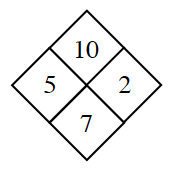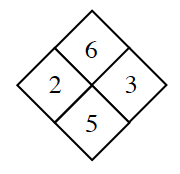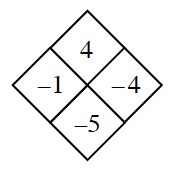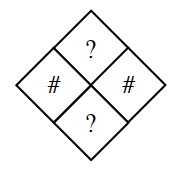Copy the Diamond Problems below onto your paper. Then use the pattern you discovered to complete each one.

1.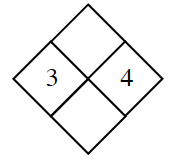1.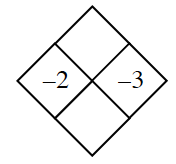1.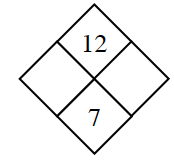1.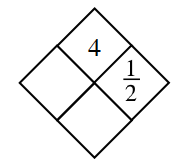1.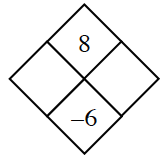What do you do to each of the # numbers to get to the ? numbers?

See the diamonds below.

In the example diamonds, notice how the two side numbers added together equal the bottom number, while those same two numbers multiplied equal the top number.